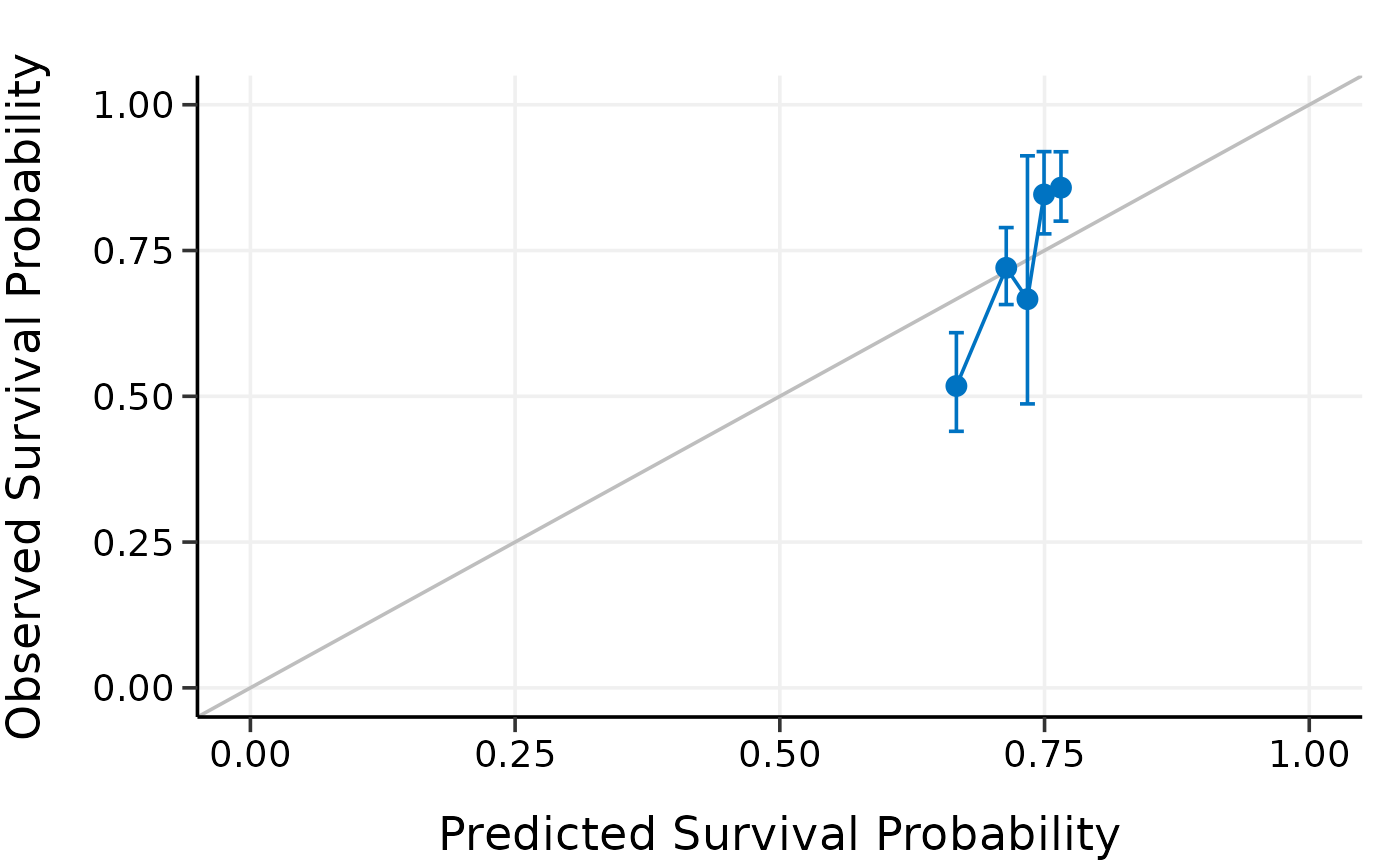Calibrate high-dimensional Cox models

calibrate(x, time, event, model.type = c("lasso", "alasso", "flasso",
"enet", "aenet", "mcp", "mnet", "scad", "snet"), alpha, lambda,
pen.factor = NULL, gamma, lambda1, lambda2, method = c("fitting",
"bootstrap", "cv", "repeated.cv"), boot.times = NULL, nfolds = NULL,
rep.times = NULL, pred.at, ngroup = 5, seed = 1001, trace = TRUE)

## Arguments

x Matrix of training data used for fitting the model; on which to run the calibration. Survival time. Must be of the same length with the number of rows as x. Status indicator, normally 0 = alive, 1 = dead. Must be of the same length with the number of rows as x. Model type to calibrate. Could be one of "lasso", "alasso", "flasso", "enet", "aenet", "mcp", "mnet", "scad", or "snet". Value of the elastic-net mixing parameter alpha for enet, aenet, mnet, and snet models. For lasso, alasso, mcp, and scad models, please set alpha = 1. alpha=1: lasso (l1) penalty; alpha=0: ridge (l2) penalty. Note that for mnet and snet models, alpha can be set to very close to 0 but not 0 exactly. Value of the penalty parameter lambda to use in the model fits on the resampled data. From the Cox model you have built. Penalty factors to apply to each coefficient. From the built adaptive lasso or adaptive elastic-net model. Value of the model parameter gamma for MCP/SCAD/Mnet/Snet models. Value of the penalty parameter lambda1 for fused lasso model. Value of the penalty parameter lambda2 for fused lasso model. Calibration method. Options including "fitting", "bootstrap", "cv", and "repeated.cv". Number of repetitions for bootstrap. Number of folds for cross-validation and repeated cross-validation. Number of repeated times for repeated cross-validation. Time point at which calibration should take place. Number of groups to be formed for calibration. A random seed for resampling. Logical. Output the calibration progress or not. Default is TRUE.

## Examples

data("smart")
x <- as.matrix(smart[, -c(1, 2)])
time <- smart$TEVENT event <- smart$EVENT
y <- survival::Surv(time, event)

# Fit Cox model with lasso penalty
fit <- fit_lasso(x, y, nfolds = 5, rule = "lambda.1se", seed = 11)

# Model calibration by fitting the original data directly
cal.fitting <- calibrate(
x, time, event,
model.type = "lasso",
alpha = 1, lambda = fit$lambda, method = "fitting", pred.at = 365 * 9, ngroup = 5, seed = 1010 )#> Start fitting ... # Model calibration by 5-fold cross-validation cal.cv <- calibrate( x, time, event, model.type = "lasso", alpha = 1, lambda = fit$lambda,
method = "cv", nfolds = 5,
pred.at = 365 * 9, ngroup = 5,
seed = 1010
)#> Start fold 1
#> Start fold 2
#> Start fold 3
#> Start fold 4
#> Start fold 5
print(cal.fitting)#> High-Dimensional Cox Model Calibration Object
#> Random seed: 1010
#> Calibration method: fitting
#> Model type: lasso
#> glmnet model alpha: 1
#> glmnet model lambda: 0.01620998
#> glmnet model penalty factor: not specified
#> Calibration time point: 3285
#> Number of groups formed for calibration: 5 summary(cal.fitting)#>   Calibration Summary Table
#>   Predicted  Observed Lower 95% Upper 95%
#> 1 0.5867665 0.4756039 0.4037162 0.5602922
#> 2 0.7013731 0.6649731 0.5400804 0.8187470
#> 3 0.7570641 0.8402387 0.7977191 0.8850247
#> 4 0.7969468 0.8009555 0.7048913 0.9101115
#> 5 0.8393141 0.9011939 0.8687962 0.9347997plot(cal.fitting)print(cal.cv)#> High-Dimensional Cox Model Calibration Object
#> Random seed: 1010
#> Calibration method: k-fold cross-validation
#> Cross-validation folds: 5
#> Model type: lasso
#> glmnet model alpha: 1
#> glmnet model lambda: 0.01620998
#> glmnet model penalty factor: not specified
#> Calibration time point: 3285
#> Number of groups formed for calibration: 5 summary(cal.cv)#>   Calibration Summary Table
#>   Predicted  Observed Lower 95% Upper 95%
#> 1 0.5838591 0.4675519 0.3945238 0.5540979
#> 2 0.7029040 0.6982853 0.5867801 0.8309796
#> 3 0.7566084 0.8315265 0.7875912 0.8779128
#> 4 0.7975700 0.7993794 0.7070266 0.9037955
#> 5 0.8387503 0.9090449 0.8776173 0.9415978plot(cal.cv)# # Test fused lasso, SCAD, and Mnet models
# data(smart)
# x = as.matrix(smart[, -c(1, 2)])[1:500, ]
# time = smart$TEVENT[1:500] # event = smart$EVENT[1:500]
# y = survival::Surv(time, event)
#
# set.seed(1010)
# cal.fitting = calibrate(
#   x, time, event, model.type = "flasso",
#   lambda1 = 5, lambda2 = 2,
#   method = "fitting",
#   pred.at = 365 * 9, ngroup = 5,
#   seed = 1010)
#
# cal.boot = calibrate(
#   x, time, event, model.type = "scad",
#   gamma = 3.7, alpha = 1, lambda = 0.03,
#   method = "bootstrap", boot.times = 10,
#   pred.at = 365 * 9, ngroup = 5,
#   seed = 1010)
#
# cal.cv = calibrate(
#   x, time, event, model.type = "mnet",
#   gamma = 3, alpha = 0.3, lambda = 0.03,
#   method = "cv", nfolds = 5,
#   pred.at = 365 * 9, ngroup = 5,
#   seed = 1010)
#
# cal.repcv = calibrate(
#   x, time, event, model.type = "flasso",
#   lambda1 = 5, lambda2 = 2,
#   method = "repeated.cv", nfolds = 5, rep.times = 3,
#   pred.at = 365 * 9, ngroup = 5,
#   seed = 1010)
#
# print(cal.fitting)
# summary(cal.fitting)
# plot(cal.fitting)
#
# print(cal.boot)
# summary(cal.boot)
# plot(cal.boot)
#
# print(cal.cv)
# summary(cal.cv)
# plot(cal.cv)
#
# print(cal.repcv)
# summary(cal.repcv)
# plot(cal.repcv)